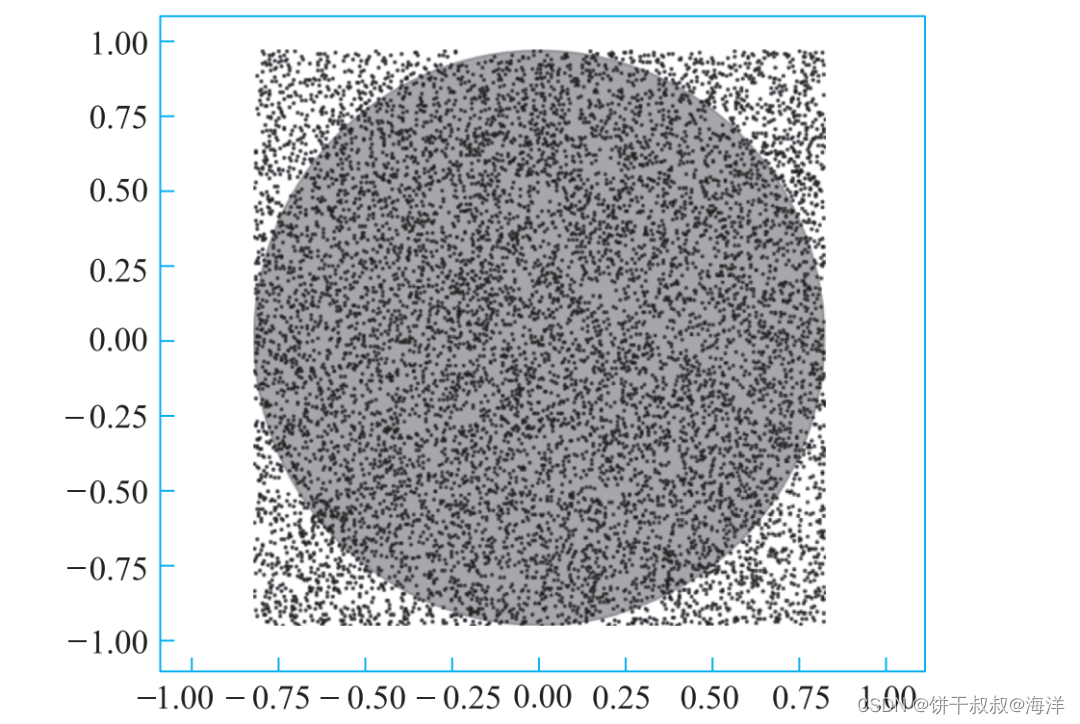# C/C++微实践 – 发现圆周率

1.《Python编程基础及应用》，陈波，刘慧君，高等教育出版社。免费授课视频 Python编程基础及应用
2.《Python编程基础及应用实验教程》, 陈波，熊心志，张全和，刘慧君，赵恒军，高等教育出版社Python编程基础及应用实验教程
3. 《简明C及C++语言教程》，陈波，待出版书稿。免费授课视频

``````//Project - FindPi
#include <iostream>
#include <cmath>
using namespace std;

int main()
{
const int N = 10000;    //投点总数
int nHits = 0;          //圆内投点数

for (int i=0;i<N;i++){
double x = 2.0*rand()/RAND_MAX - 1.0; //随机数取投点x坐标
double y = 2.0*rand()/RAND_MAX - 1.0; //随机数取投点x坐标
if (x*x + y*y <= 1.0)  //投点位于内切圆内
nHits += 1;        //圆内投点数+1
}

double pi = 4.0*nHits/N;   //通过计算圆面积估算圆周率
cout << "pi = " << pi;
return 0;
}
``````

``````pi = 3.1464
``````

Native

TCP与UDP

1024程序员节

iVXPython编程基础及应用Python编程基础及应用

KMM

Python编程基础及应用实验教程progressbar